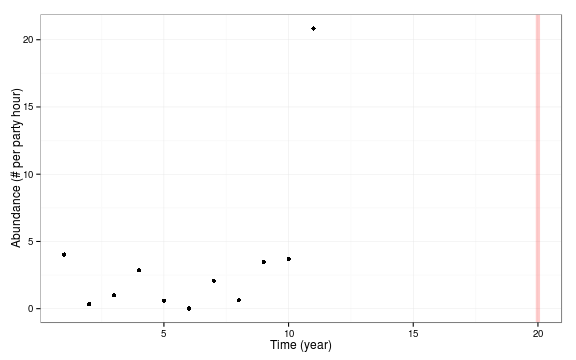# Fitting logistic models to initial data

## Introduction

This question on Stack Overflow discusses the difficulty of fitting logistic models using standard tools in R. The examples are admittedly ones where the logistic model is completely bogus (i.e., the temporal patterns show a marked peak and then a decline, not something that can be well represented by a standard logistic), but the data still make a reasonable example for computationally difficult numerical optimization problems.

I initially thought the problem was one of estimating a logistic just from exponential-phase data, which is closely related to the problem posed in the “weeds” project writeup at this site. In this case, the problem is difficult because the asymptote is very poorly constrained by the data. It is even harder if one uses a parameterization in which the value of the asymptote also affects the parameters used to estimate the other aspects of the growth curve (initial growth rate, inflection point). However, in the actual example given, the cutoff in time for the optimization is somewhat past the inflection point, so the problem isn't actually that hard.

## Cattle egret

### Data

CEdat <- data.frame(time = 1:45, obs = c(0.3061324, 1e-05, 0.2361211, 0.505824,
2.0685032, 2.1944544, 4.2689494, 4.9508297, 3.133472, 3.6570752, 5.6753381,
10.9133183, 5.4518257, 20.4166979, 15.9741054, 19.0970426, 13.7559959, 14.1358153,
15.9986416, 29.6762828, 10.3760667, 8.4284488, 6.1060359, 3.7099982, 3.358406,
2.5981386, 2.5697082, 2.8091952, 5.5487979, 1.6505442, 2.2696972, 2.1835692,
3.6747876, 4.8307886, 3.5019731, 2.8397137, 1.8605288, 11.1848738, 2.6268683,
4.1215127, 2.399621, 2.6569938, 2.1987387, 3.0267252, 2.4420927))


Initial plot, with cutoff at $$t=20$$ shown:

library(ggplot2)
theme_set(theme_bw())
(g0 <- ggplot(CEdat,aes(x=time,y=obs))+geom_point()+
labs(y="Abundance (# per party hour)",x = "Time (year)")+
geom_vline(xintercept=20,lwd=2,
colour="red",alpha=0.2))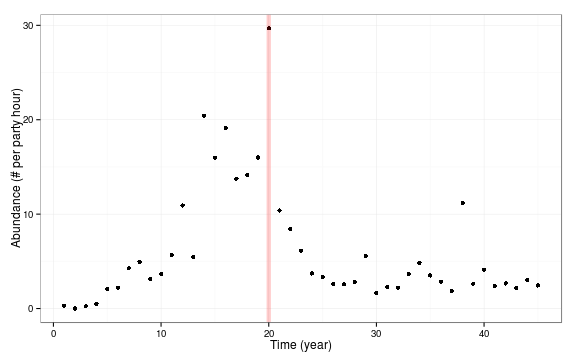### Model fits

We can fit the model to the initial data reasonably easily with the self-starting logistic (SSlogis) function:

CE.mod <- nls(obs ~
SSlogis(time, Asym, xmid, scal),
data=subset(CEdat, time<=20))


Trying to fit the full data set fails:

update(CE.mod,data=CEdat)

## Error: step factor 0.000488281 reduced below 'minFactor' of 0.000976562


We fail even if we just try to retrieve the initial values from SSlogis():

getInitial(obs~SSlogis(time,Asym,xmid,scal),data=CEdat)

## Error: step factor 0.000488281 reduced below 'minFactor' of 0.000976562


This fails inside stats:::getInitial.selfStart, where it tries to use nls with algorithm="plinear" model to fit a logistic with initial coefficients based on a logit fit to the data. Specifically:

x <- CEdat$time z <- CEdat$obs
z <- z/(1.05 * max(z))  ## scale to max=1/(1.05)
zlogit <- log(z/(1-z))
cc <- setNames(coef(lm(x~zlogit)),c("a","b"))


This is the linear function that SSlogis() fits (!!)

qplot(zlogit,x)+geom_smooth(method="lm",se=FALSE,colour="red")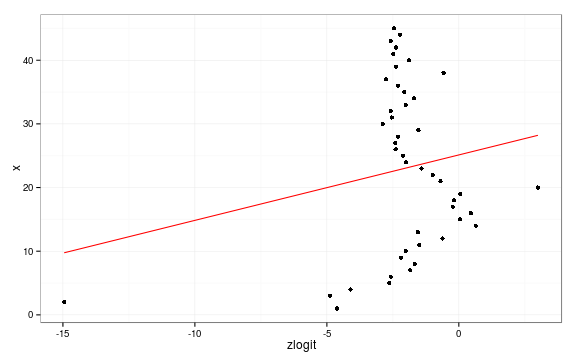And this is the corresponding logistic fit:

predfun <- function(x)
with(as.list(cc),
plogis((x-a)/b)*1.05*max(z))
g0+stat_function(fun = predfun,colour="red")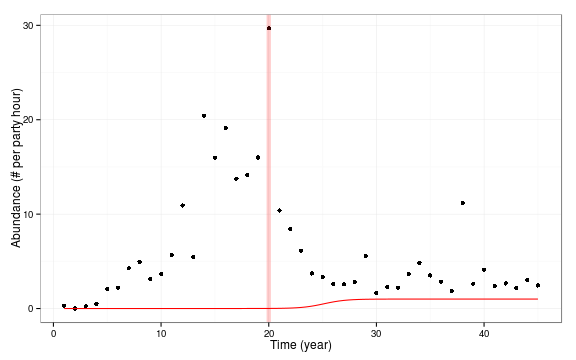Perhaps not so surprising that it fails.

If we pick more plausible starting values for this example (asymptote=max value, midpoint = midpoint of the data series, scale=1), then we do fine:

newstart <- list(Asym = max(CEdat$obs), xmid = mean(CEdat$time),
scal=1)
CE.fullmod <- update(CE.mod,data=CEdat,
start=newstart)


Results:

summary(CE.fullmod)

##
## Formula: obs ~ SSlogis(time, Asym, xmid, scal)
##
## Parameters:
##      Estimate Std. Error t value Pr(>|t|)
## Asym     7.07       1.05    6.76  3.2e-08 ***
## xmid     7.19       2.56    2.80   0.0076 **
## scal     1.42       2.25    0.63   0.5309
## ---
## Signif. codes:  0 '***' 0.001 '**' 0.01 '*' 0.05 '.' 0.1 ' ' 1
##
## Residual standard error: 6.02 on 42 degrees of freedom
##
## Number of iterations to convergence: 33
## Achieved convergence tolerance: 8.1e-06

logLik(CE.fullmod)

## 'log Lik.' -143.1 (df=4)


The minpack.lm:::nlsLM function does no better or worse (since the failure point is in the self-starting function itself, not in the fitting function) [not shown].

getpred <- function(model=CE.mod,times=CEdat$time) { xdata <- data.frame(time=times) xdata$obs <- predict(model, newdata=xdata)
xgrad <- attr(xdata$obs, "gradient") ## p1 and p2 are equivalent computations ## of confidence intervals; I think p2 ## is a little more general ## p1 <- sqrt(apply(xgrad, 1, function(x) ## sum(vcov(CE.mod)*outer(x,x)))) p2 <- sqrt(pvar2 <- diag(xgrad %*% vcov(CE.mod) %*% t(xgrad))) ## prediction intervals p3 <- sqrt(pvar2 + summary(CE.mod)$sigma^2)
data.frame(xdata,
lwr=xdata$obs-1.96*p2, upr=xdata$obs+1.96*p2,
lwr2=xdata$obs-1.96*p3, upr2=xdata$obs+1.96*p3)
}
xdata <- getpred(CE.mod)
xdata.full <- getpred(CE.fullmod)

g0 + geom_line(data=xdata)+
geom_ribbon(data=xdata,
aes(ymin=lwr,ymax=upr),alpha=0.2)+
geom_ribbon(data=xdata,
aes(ymin=lwr2,ymax=upr2),alpha=0.2)+
geom_line(data=xdata.full,colour="red")+
geom_ribbon(data=xdata.full,
aes(ymin=lwr,ymax=upr),alpha=0.2,fill="red")+
geom_ribbon(data=xdata.full,
aes(ymin=lwr2,ymax=upr2),alpha=0.2,fill="red")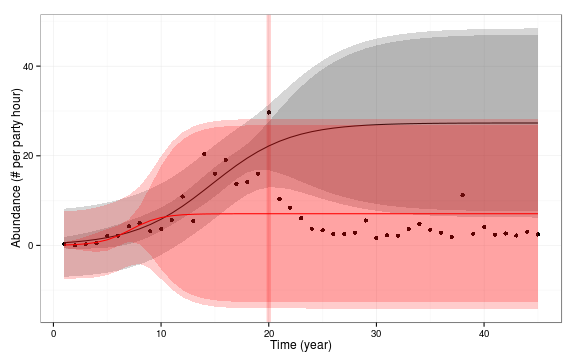## Chestnut manikin

ChMa <- data.frame(obs = c(4.02785074, 0.33847154, 0.99029776, 2.8651654, 0.59588068,
0.01334333, 2.07693362, 0.62485994, 3.48979515, 3.67785202, 20.84180181),
time = 1:11)


Are these data worth doing anything with at all??

g0 %+% ChMa